# Measuring Current/Voltage with arduino ?

Hi Guys,

Would it be possible to be measure voltage and current with my arduino using the analog input pins? I'd like to measure a voltage between 0-9V, and a current between 0 and 30mA. The numbers aren't too extreme so would this be possible without using any other special ic's ?

The arduino analog input pins can by default meassure 0 - 5V with 10 bit resolution i.e it will give you a number in the 0 - 1023 range. You can scale the 0 - 9 V voltage down to "fit" in the 0 - 5 volt range with a simple OpAmp circuit or a voltage divider maybee.

I don't know about meassuring current with arduino. But it should be possible to actually convert a current to a voltage. Try to Google "current to voltage conversion"

Remember to consider your needed precision. How precise do yuor meassurements have to be?

Well, it doesn't have to be too precise but I would like to get values that make sense. I'll have a look for a current to voltage conversion. Anybody else have any ideas ?

Hi Guys,

Would it be possible to be measure voltage and current with my arduino using the analog input pins? I'd like to measure a voltage between 0-9V, and a current between 0 and 30mA. The numbers aren't too extreme so would this be possible without using any other special ic's ?

The voltage divider suggestion will work to measure voltage.

For current, you would use a resistor as a shunt and measure the voltage across the resistor.

OK, so this is what I've got so far:

For my current input, I can basically use a resistor (as mentioned above) in the following setup: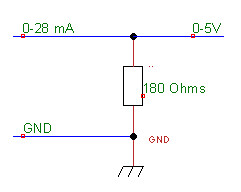From my calculations (V=IR) I get that at 27.7mA my output would be 5v. So theoretically I can just measure my output with the arduino analog input and then do some calculations to get the mA.

The voltage divider is easy enough :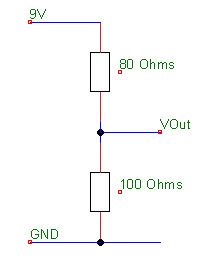Which means that I should get 5V out with a 9V input. To calculate Vin I use the following formula:

Vin = Vout/(R2/(R1+R2))

Am I correct with these assumptions or do you guys disagree with something. btw - I know the theory (supposedly ;)), but I'm still learning to apply this practically.

With that 180 ohm resistor you'll be losing 5 volts of your total voltage available to the circuit. Can you afford to lose that much?

Your voltage divider will consume 50ma of current. This is way too much. Bump your resistances up by a factor of 10 and only consume 5ma. Also note that 80 ohms is not a standard value. 82 is though.

I suggest using even higher resistors in the voltage divider. If you use two 4.7k resistors than the voltage range will be 0-10 volts. Dividing the analogRead value by 102 you will give you the result in volts. (dividing by 102.4 is slightly more accurate but uses floating point which makes your program much bigger)

You want the smallest possible resistor for measuring the current, but as the resistor gets smaller the accuracy drops. If you use a 1 ohm resistor then each unit of analogRead of the pin connected to the current sensing resistor is around 5ma. A 5.1 ohms resistor gives around 1ma for each unit of analogRead.

You want the smallest possible resistor for measuring the current, but as the resistor gets smaller the accuracy drops. If you use a 1 ohm resistor then each unit of analogRead of the pin connected to the current sensing resistor is around 5ma. A 5.1 ohms resistor gives around 1ma for each unit of analogRead.

OK, so i've got something setup on my breadboard. From the calculations I should be reading 25.5 mA. I used a 4.7 ohms resistor for measuring the voltage from. At the moment the arduino board analog reading is jumping between 23 and 24, which is pretty damn close. How did you calculate that 5.1 ohms will give you around 1ma for each unit read. ie. how can I convert that value to get an even more accurate value. I'm guessing that using precision resistors will give me an even more accurate value ?

thanks for all the help so far everybody, I really appreciate it.

An analogRead value of 1024 equals 5 volts, so each unit equals: 5 divided by 1024, which is 4.9 millivolts.

By Ohms law, the voltage drop on a resistor equals the amps times resistance in ohms. Therefore 4.9 millivolts will be the voltage drop across a resistor of 4.9 ohms when the current is 1 milliamp .

Standard resistor values are 4.7 ohms or 5.1 ohms but you can get exactly 4.9 ohms using a 5.1 ohm and 120 ohm resistor in parallel. Using this, the value you get from analogRead will be the current in milliamps.

hmm, I ran into a problem. I've got a circuit with a current regulator and a led. I'm measuring the current by adding the 4.7ohm resistor in series and measuring the voltage over it with my arduino. That part seems to be working fine, but now I also want to measure the voltage over the led using the same arduino. If I take a wire from ground to the one side of the led and and the other side of the led to another analog input to measure it, it seems to short out the led. I hope this made sense ...

Can I measure the voltage over the led with the same setup ?

analogRead measures the voltage potential between a pin and ground. If you want to measure the voltage with reference to a voltage above ground, you could take two measurements, one on each LED lead and subtract the difference.

Just a reminder - measure your resistor and use it's actual value when calculating current - the difference between marked value and actual can be quite large.

If I take a wire from ground to the one side of the led and and the other side of the led to another analog input to measure it, it seems to short out the led. I hope this made sense ...

Can I measure the voltage over the led with the same setup ?

Yes - you should be able to. Make sure both inputs are configured as inputs.

Mike

Thanks for all the help so far guys, but at the moment I'm a bit confused:

This is the original circuit :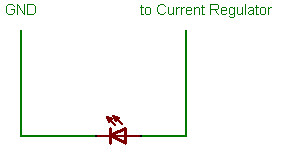So now I wanted to measure the current so I added the resistor and read the voltage value with the arduino analog input like so :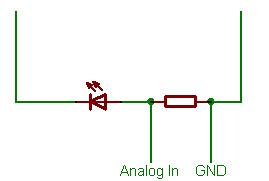OK, that works nicely, But, now I also want to measure the voltage over the led : But obviously I can't just add another ground before the led and a an arduino pin after the led. So do I just set GND to starting ground and then read voltages at the different points with multiple analog inputs ? :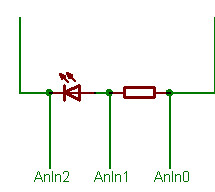does that make sense ? Will my 'current measure resistor' still do its job that way?

Thanks again for all the help

Your ground got switched around between the first and second diagrams.

+v via current regulator
|
|----- analog2 in (LED anode)
|
\ / <- LED
v
|
------- analog1 in (LED cathode)
|
[ ]
[ ] <- current sensing resistor
[ ]
|
------------ Ground

analog1 value will be proportional to current

analog2 will be the voltage on the led anode

(analog2 - analog1) value will be the voltage across the diode

Thanks, I'll give it a shot tonight and see how it works. Thanks for everybodies help and especially MemOnce you get the voltage/current in the Arduino,

Here is a video displaying it on an OLED,
http://www.liquidware.org/view.php?id=76&phase=idea

I kinda wanna do the same. I have a rc el glider with an brush less motor with regulator. I would like to measure the current, voltage and rpm of the motor. The output effect is around 600W, which yields that the voltage divider needs to scale down 3 Lipo cell = 12 voltage, and around 60 amp for the current.
Is it possible to connect one wire to one of the 3 wires of the regulator to measure the rpm?
Any suggestion of how I can do this?

Kim Skatun

Repeating the (unanswered) question above: I would like to have a 3.3V Arduino board on a vehicle, and the current to be measured is up to 120 amps for about 22 volts.

I understand the idea of a shunt, but I don't know how to scale up the concept to deal with the circuit. Of course, I also don't want to burn up much power in the measurement function.

halley:

you would need a really accurate, really small resisitor. The main circuit has a resistance of 0,18 Ohm, if you make a shunt 1/100th f that it will have a voltage drop of 0,22V. But that means a shunt of 0,0018 Ohm. I see 2 ways you could try.

1- take 50 meter of copper wire (i would take my roll of extension cord, though I'm not sure that could take 120A) . Measure the resistance of this ( say that's 2,0 Ohm) Then make a linear interpolation and cut a short piece from the wire. Use this as resistor (in my example, this would be a piece of 4,5 cm)
Now, this would still give 120A*0,22V=26W ! of heat dissipation in this wire, which is probably too much. And if the wire gets hot, the resistance will change... Hm. Cutting the short wire in 2 isn't very practical, so lets use 10 equally sized pieces in parallel to build the shunt. This divides the resistance by 10, voltage drop is now 0,022V.

You can use the above multiplying method to build a 'radiator' of wires and build op a resistor that has a small resistance and enough surface to be cooled.

Also, if you can get your hands on a spool of constantan wire, that's very good to make resistors from. This is an alloy designed to have the same resistance as temperature increases.

Method 2: For this method you need an Amp-meter that can measure 120 Amp (mine sure can't)
Just use a piece of the regular wire in the circuit as shunt. Measure the voltage across this wiie with the circuit described above while you place the Amp-meter in the circuit. You can now see what voltage drop across this wire corresponds to what Amperage. Basically, if you have an amp-meter, you can build onehope this helps,
Lieven